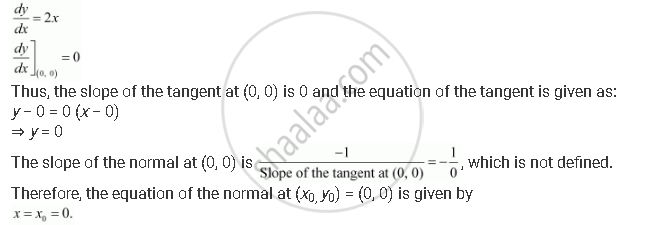Share

# Find the Equations of the Tangent and Normal to the Given Curves at the Indicated Points: Y = X2 At (0, 0) - CBSE (Science) Class 12 - Mathematics

#### Question

Find the equations of the tangent and normal to the given curves at the indicated points:

y = x2 at (0, 0)

#### Solution

The equation of the curve is y = x2.

On differentiating with respect to x, we get:Is there an error in this question or solution?

#### APPEARS IN

NCERT Solution for Mathematics Textbook for Class 12 (2018 to Current)
Chapter 6: Application of Derivatives
Q: 14.4 | Page no. 212

#### Video TutorialsVIEW ALL 

Solution Find the Equations of the Tangent and Normal to the Given Curves at the Indicated Points: Y = X2 At (0, 0) Concept: Tangents and Normals.
S